# The Laplacian matrix¶

In this homework, you will learn about the Laplacian matrix of a graph.

Recall that from a graph $G$ with $n$ nodes, we can produce the following $n \times n$ matrices:

• the adjacency matrix $A$
• the degree matrix $D$, a diagonal matrix with degrees of nodes along the diagonal
• the random walk matrix $W = AD^{-1}$

The Laplacian matrix is another $n \times n$ matrix, defined as: $$L = D - A$$

The networkx package provides a function for computing the Laplacian matrix of a graph:

In :
import networkx as nx
import numpy as np
np.set_printoptions(formatter={'float': lambda x: "{0:0.2f}".format(x)})

G = nx.Graph([(1,2),(1,3),(2,4),(1,5), (2,3), (4,5), (2,5)])
nx.draw(G,  with_labels = True, node_color = 'white')

nx.laplacian_matrix(G).todense()

Out:
matrix([[ 3, -1, -1,  0, -1],
[-1,  4, -1, -1, -1],
[-1, -1,  2,  0,  0],
[ 0, -1,  0,  2, -1],
[-1, -1,  0, -1,  3]], dtype=int32)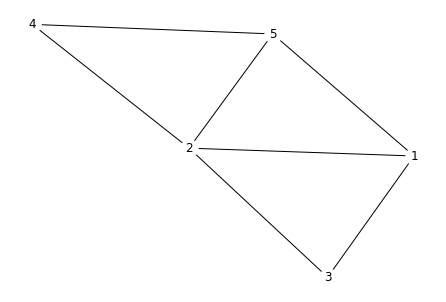## Factoring the Laplacian¶

The Laplacian matrix is symmetric (because $A$ and $D$ are symmetric). But more than that, it can actually be expressed as

$$L = M M^\top$$

for some matrix $M$.

The matrix $M$ is the signed (or oriented) incidence matrix, which is an $n \times m$ matrix where $m$ is the number of edges of $G$.

Each column of $M$ represents an edge. If $e$ is an edge between nodes $i$ and $j$, with $i < j$, we put:

• $-1$ in the $i$th entry of column $e$,
• $1$ in the $j$th entry, and
• zeroes elsewhere

This sounds complicated, so let's just compute an example. Luckily, networkx provides us the incidence_matrix function. Look at the output one column at a time, and try to make sense of the definition:

In :
M = nx.incidence_matrix(G, oriented = True)
M = M.todense().astype(np.int)
M

Out:
matrix([[-1, -1, -1,  0,  0,  0,  0],
[ 1,  0,  0, -1, -1, -1,  0],
[ 0,  1,  0,  0,  1,  0,  0],
[ 0,  0,  0,  1,  0,  0, -1],
[ 0,  0,  1,  0,  0,  1,  1]])

Now let's check that $MM^\top$ gives us $L$:

In :
M @ M.T

Out:
matrix([[ 3, -1, -1,  0, -1],
[-1,  4, -1, -1, -1],
[-1, -1,  2,  0,  0],
[ 0, -1,  0,  2, -1],
[-1, -1,  0, -1,  3]])

We won't actually use the matrix $M$. We only care that $L$ can be factored as $M M^\top$. Not all symmetric matrices can be expressed in this way (for example, $A$ cannot always be expressed as $N N^\top$ for some $N$).

## Question 1¶

We now know that $L = MM^\top$. Let $v$ be an eigenvector of $L$ with eigenvalue $\lambda$.

($v^\top$ represents $v$ treated as a row vector)

a) Write an expression for $v^\top L v$ in terms of $v$ and $\lambda$.

b) Write an expression for $v^\top L v$ in terms of $M^\top v$.

c) Show that this implies that $\lambda \geq 0$.

This is sort of like how if $x = y^2$ for some real number $y$, then $x \geq 0$.

## Question 2¶

We've seen that all eigenvalues of $L$ are at least $0$. We will now show that $0$ is in fact an eigenvalue (hence the smallest eigenvalue of $L$).

Show that the vector of all-ones $\begin{pmatrix} 1 \\ 1 \\ \vdots \\ 1 \end{pmatrix}$ is an eigenvector of $L$. (Hint: recall how we defined the degree vector $d$ and the degree matrix $D$)

What is the corresponding eigenvalue?

# Computing the Laplacian spectrum¶

By now, you should be able to write your own function for computing the eigenvalues of the Laplacian, by applying np.linalg.eigvalsh to the Laplacian matrix.

But you don't have to do it: the eigenvalues of the Laplacian are so important that networkx includes a function just for computing it!

Let's see if it's indeed true that $0$ is the smallest eigenvalue:

In :
nx.laplacian_spectrum(G)

Out:
array([-0.00, 1.59, 3.00, 4.41, 5.00])

# Disconnected graphs¶

The graph $G$ above was a connected graph. Suppose now that we have a disconnected graph $H$, such as the following:

In :
H  = nx.Graph([(1,2),(1,3),(2,4),(1,5), (2,3), (6,7),(6,8),(7,8),(8,9)])
nx.draw(H,  with_labels = True, node_color = 'white')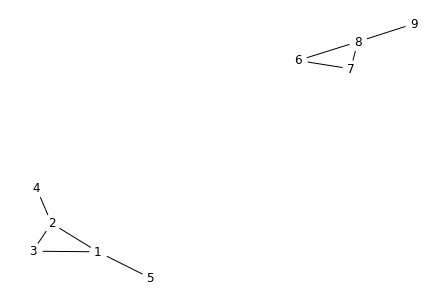Let's compute the Laplacian spectrum of $H$:

In :
nx.laplacian_spectrum(H)

Out:
array([0.00, 0.00, 0.70, 1.00, 1.38, 3.00, 3.62, 4.00, 4.30])

Notice that the second eigenvalue is now $0$: this is not a coincidence.

Since $H$ is disconnected, its adjacency matrix can be written in the form

$$A = \begin{pmatrix} A_1 & 0 \\ 0 & A_2\end{pmatrix}$$

where $A_1$ and $A_2$ are adjacency matrices of the connected subgraphs $H_1$ and $H_2$ of $H$, and the $0$s stand for matrices of all zeros.

The degree and Laplacian matrices can also be expressed in a similar fashion.

## Question 3¶

Show that $\begin{pmatrix} 1 \\ 1 \\ \vdots \\ 1 \\ -1 \\ -1 \\ \vdots \\ -1 \end{pmatrix}$ is an eigenvector of the Laplacian of $H$, where the number of positive ones is the same as the number of nodes in $H_1$.

What is it's eigenvalue?

## Challenge Question¶

This question is not compulsory.

You have shown that if a graph is disconnected, then $\begin{pmatrix} 1 \\ 1 \\ \vdots \\ 1 \\ -1 \\ -1 \\ \vdots \\ -1 \end{pmatrix}$ is an eigenvector with eigenvalue $0$.

Show that the converse is true: if this vector is an eigenvector, then the graph is disconnected.

# The algebraic connectivity of a graph¶

In fact, even more is true, although we will not show it:

Theorem. Let $G$ be a graph, and $L$ its Laplacian matrix. The second smallest eigenvalue $L$ is $0$ if and only if $G$ is a disconnected.

So if we write the eigenvalues of $L$ as $0 = \lambda_1 \leq \lambda_2 \leq \dots \leq \lambda_n$, we can tell if $G$ is connected just by looking at $\lambda_2$.

Because of this, $\lambda_2$ is called the algebraic connectivity of a graph.

Instead of just seeing whether $\lambda_2$ is $0$ or not $0$, we can also ask how big $\lambda_2$ is. It turns out that this behaves in a nice way:

Theorem. Let $G$ be a graph, and $H$ be another graph containing all the nodes and edges of $G$ but with additional edges. Then $\lambda_2$ of $H$ is larger than $\lambda_2$ of $G$.

We won't prove these theorems, but let's look at the second smallest eigenvalue of the following graphs to see that they are true.

In :
P = nx.path_graph(10)
print(nx.laplacian_spectrum(P))
nx.draw(P, with_labels = True, node_color = 'white')

[0.00 0.10 0.38 0.82 1.38 2.00 2.62 3.18 3.62 3.90]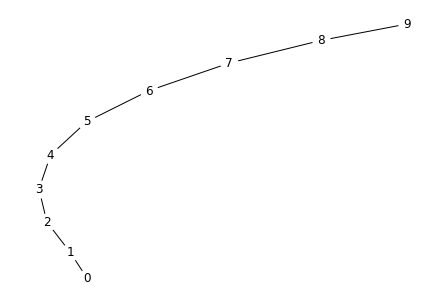In :
B = nx.barbell_graph(4,2)
print(nx.laplacian_spectrum(B))
nx.draw(B, with_labels = True, node_color = 'white')

[-0.00 0.14 1.00 2.68 4.00 4.00 4.00 4.00 5.00 5.18]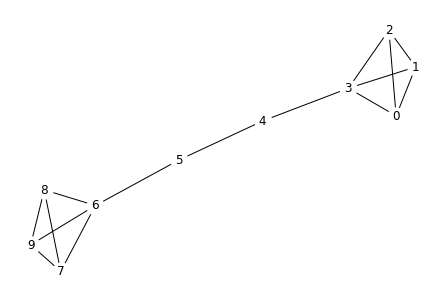In :
C = nx.cycle_graph(10)
print(nx.laplacian_spectrum(C))
nx.draw(C, with_labels = True, node_color = 'white')

[0.00 0.38 0.38 1.38 1.38 2.62 2.62 3.62 3.62 4.00]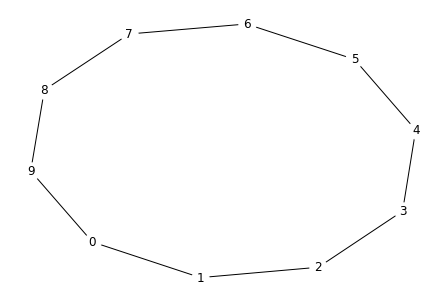In :
S = nx.star_graph(9) # nx.star_graph(n) returns a graph with n+1 nodes
print(nx.laplacian_spectrum(S))
nx.draw(S, with_labels = True, node_color = 'white')

[-0.00 1.00 1.00 1.00 1.00 1.00 1.00 1.00 1.00 10.00]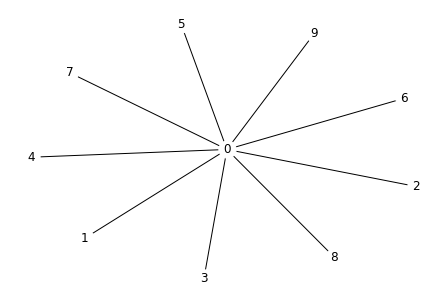In :
Wh = nx.wheel_graph(10) # I'm calling this Wh because we already used W for the walk matrix
print(nx.laplacian_spectrum(Wh))
nx.draw(Wh, with_labels = True, node_color = 'white')

[0.00 1.47 1.47 2.65 2.65 4.00 4.00 4.88 4.88 10.00]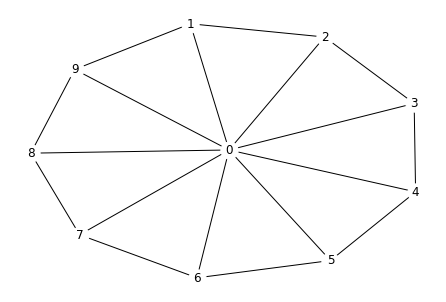In :
K = nx.complete_graph(10)
print(nx.laplacian_spectrum(K))
nx.draw(K, with_labels = True, node_color = 'white')

[0.00 10.00 10.00 10.00 10.00 10.00 10.00 10.00 10.00 10.00]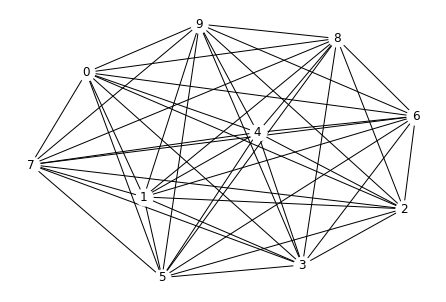Does the above ranking agree with your intuition on how connected the graphs are?

Observe that according to this standard, the barbell graph is now more connected than the path graph.

However, note that the cycle graph is more connected than the barbell graph. Does this make sense to you?

In the barbell and cycle graphs, you can get anywhere on the graph within 5 steps. So one might expect that these two graphs have similar connectivity.

But let's take a different approach: how easy is it to disconnect a graph by removing an edge?

For the barbell graph, we can remove a single edge (e.g. between nodes 4 and 5) to disconnect it. But for the cycle graph, we need to remove 2 edges to disconnect it. So from this perspective, the cycle graph should be quite a bit more connected than the barbell graph, and this is reflected in its second smallest eigenvalue.